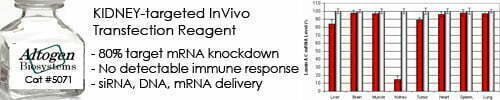••••Shop Products

# Moles of ssDNA ends Calculator

The Moles of ssDNA ends calculator is a tool that calculates the number of moles of single-stranded DNA (ssDNA) ends based on the amount of ssDNA in moles and the number of ends present. This calculation is important in various applications in molecular biology and biotechnology, such as cloning, sequencing, and gene expression analysis.

The formula for calculating the moles of ssDNA ends is:

moles of ssDNA ends = (moles of ssDNA x ends per molecule) / 2

Where:

• moles of ssDNA is the amount of ssDNA in moles
• ends per molecule is the number of free ends present in each ssDNA molecule
• The division by 2 is because each double-stranded DNA (dsDNA) molecule has two ends

To use the Moles of ssDNA ends calculator, you need to input the values of moles of ssDNA and the number of ends per molecule, and the calculator will calculate the corresponding number of moles of ssDNA ends. The resulting mole value can be useful in various applications in molecular biology and biotechnology, such as determining the amount of ssDNA ends needed for a particular experiment or reaction.

It is important to note that the number of ends per molecule may vary depending on the type of ssDNA, the method of preparation, and the specific application, and that this value should be carefully determined or estimated in each case.

Moles of ssDNA ends calculator:
Enter DNA length:
nt
Enter DNA mass:
μg

Sorry, comments are closed for this post.